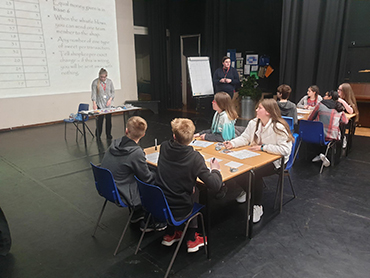Mathematical Sciences at Leicester

# Sample talks for schools

## Maths and what it can do for you

I will discuss why the study of mathematics can be so rewarding, both intellectually and financially. Using my own career journey by way of example, you will hear how a mathematical education is a great career investment in an exciting and rapidly-changing world that is increasingly dominated by the digital domain. The talk ends with some words of advice for entering university, no matter which subject you choose to follow (although we do hope it’s maths!).

## The beauty of shapes - encounters with the weird world of topologyI will be guiding you on a visual expedition into the weird world of topology, which deals with classifications of abstract geometrical shapes in many dimensions. You will see how, using mathematics, we can find ways to orient ourselves in this strange world and classify all the weird shapes we encounter. You will meet lots of interesting polyhedra, tasty doughnuts, one-sided paper stripes, weird bottles, beautiful surfaces given by algebraic equations and many other animals from the topological zoo.

## Chaos and fractals

I will introduce the concept of 'chaos' and how it leads to very complicated geometric structures called 'fractals'. Fractals are very peculiar and have unusual properties. For example, a fractal can contain as many points as an interval, yet have no length. Also, the dimension of a fractal is not 1 (like a line), 2 (like area), or 3 (like volume), but somewhere in between. Finally, I will give you a recipe for how to construct fractals and you can try to construct your own.

## Euler's formula

In this elementary talk, we discuss the beauty of polyhedral shapes and how to classify them using a simple counting trick via Euler's formula.

## Juggling mathematics

Learn to juggle by numbers, with one, two or four hands, with or without the help of the computer. We introduce the mathematical language used by jugglers worldwide to communicate, model and develop their routines since the 1980s. The workshop will include hands-on practical sessions, both real and virtual. Design your own original juggling patterns and put them into practice!

## Statistics, beauty, the golden ratio and mathematical analysis

In this talk, we will look at the average ratio of proportions of the human face, and how many we need in a sample to get a good approximation to an average. We will then look at a puzzle and see how the solution to the puzzle relates to the ratio above. Finally, we will look at how to compute this ratio as the limit of a sequence.

## The chances of finding Richard III

Assessing how likely it was that we would find the remains of Richard III at the point at which it was decided to go ahead with the excavation, using a Bayesian probability approach.

## Mathematical puzzles and games

Many mathematicians become interested in mathematics through games and puzzles. In this talk I will introduce some games and puzzles which require particular forms of mathematical thinking to solve. It will be in workshop format with lots of interaction. Students will need to bring a pen and some paper.

## Dirichlet's principle: what have hairs to do with pigeonholes?

We will show that obvious statements like "if you have fewer pigeon holes than pigeons and you put every pigeon in a pigeon hole, then there must result at least one pigeon hole with more than one pigeon" can be formalised into a powerful counting argument, Dirichlet's principle, with lots of surprising applications in mathematics and daily life.

## Mathematics and how to make your first million

Mathematicians that have solved difficult and long-standing problems have been honoured not only with immense respect from their colleagues, but also with worldwide fame. I will talk about the story of one mathematician who solved one of the most famous maths problems, which, had he solved it some years later, could possibly have won him a \$1,000,000 prize. I will finish with 7 other unsolved problems that could make a millionaire of the people who solve one of them.